# Cost rates

The screenshots on this page do not all reflect the new web version yet.

Cost rates are relevant when you add new members to the list of costs at the Activity List as well as when you add a cost to a case, case estimate or estimate template.

Usually you set the cost rate that you want used for the cost at the Activity List.

When a cost is added to a case, case estimate or estimate template, the rate defined in the Activity List applies. You can only alter this rate if you have the permission Can alter rate when entering costs.

When you change this rate in an estimate or estimate template and costs are added at a case that are linked to the estimate, this new rate applies.

You can also set specific company rates that override this rate, see Cost rate settings below.

## Rates and different kinds of costs

For the three types of costs, cost rates are relevant in different ways.

### Activity costs

For Activity costs the rate refers to an hourly rate:

When such a cost is added it has a Rate (A), which is the hourly rate for the activity.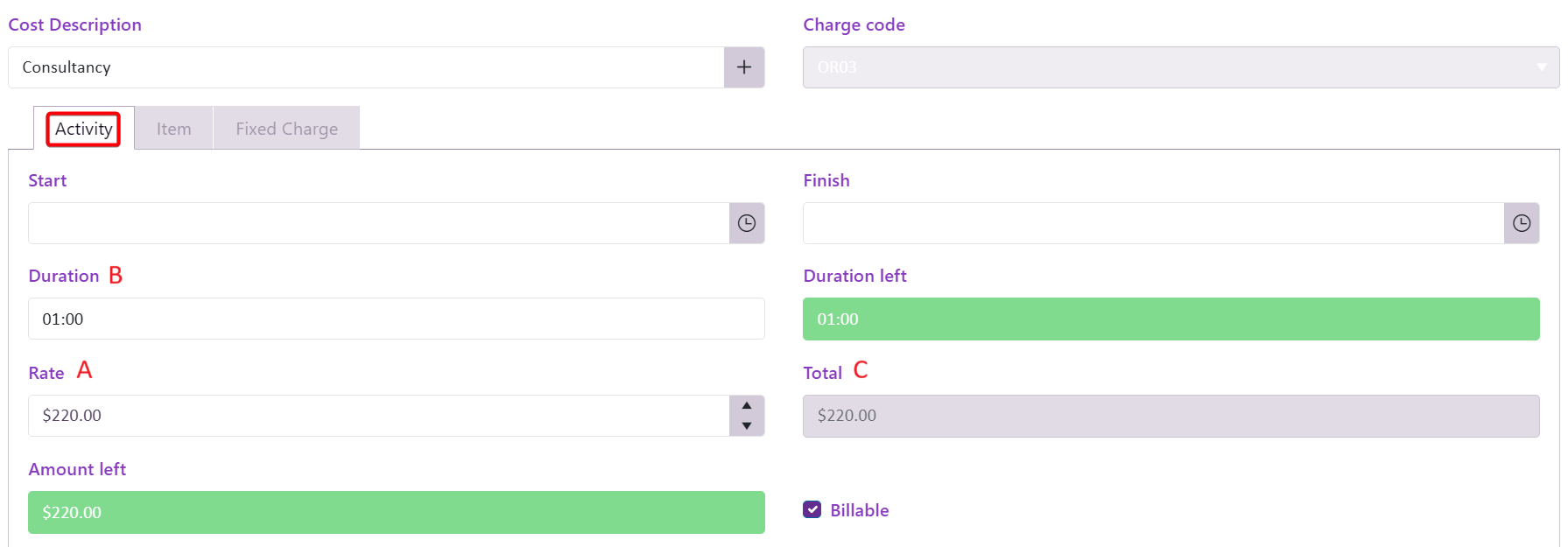The total cost (C) is calculated by multiplying this rate by the Duration (B), i.e. the time spent on this activity.

The rate that appears here is customisable and can be set at three levels, see below.

It can also be changed on this screen, subject to the user having the Can alter rate when altering costs permission: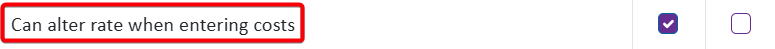### Item costs

For Item costs, the rate refers to the unit rate, i.e. the cost per item. The total cost (C) is calculated by multiplying this rate (A) by the Quantity (B), i.e. the number of items.The rate that appears here is also customisable and can be set at two levels, see below. It can also be changed on this screen, subject to the user having permission.

### Fixed Charge costs

For Fixed Charge costs the rate refers to the total rate of the charge. When a cost is added the Duration (B) is entered, as for Activity costs above, but the total cost (C) is uninfluenced by this and is calculated only from the cost rate (A). Thus, here the rate represents the entire value of the cost.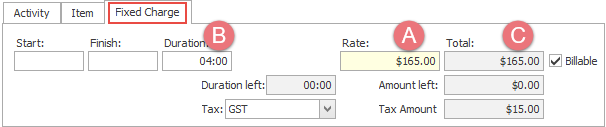The rate that appears here is also customisable and can be set at the category and system levels, see below. It can also be changed on this screen, subject to the user having permission.

## Cost rate settings

Cost rates are set at three levels :

### Company level

A fixed hourly rate, a discount or a increased cost can be set at a company level.

The company rate applies to all Activity costs added to a case or case estimate when the company is the case's Bill To contact. This rate does not apply to Fixed Charge costs or Item costs. You set the rate at the Company Settings tab for the company in the Company List, see Company settings: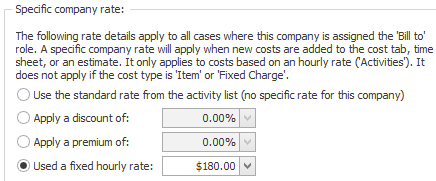This company rate does not apply to individual Activity costs when their Prevent company rate checkbox is ticked at the Activity List.### Category level

The next most important setting is at the category level. There are two relevant settings:

• You can specify in the Costs tab of the category settings that any company rates (above) do not apply to a case when it has this category, see Category settings.
•• You can set the default category rate at the Accounting tab of the category settings, see Category settings
•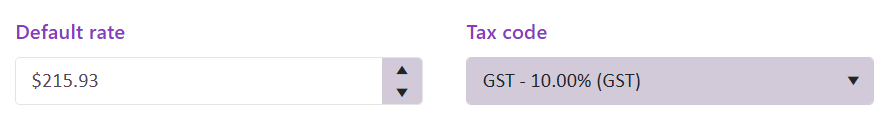This applies:

• when an estimate 'polices' via charge codes and contains charge codes.
• The rates start off with the category's default rate, which can of course be modified.

• when users are permitted by another category setting to enter any new costs to a case (i.e. costs not already in the Activity List):
•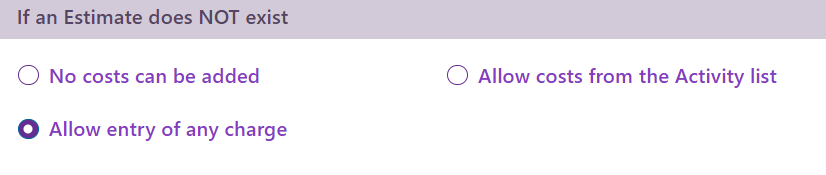This category setting is at the Costs tab, see Category settings.

• when you create a new cost at the Activity List and it is linked to one category.
• The new cost starts off with the category's default rate, which can of course be modified.

### System level

The system has a default rate that is used for costs whose rates are not determined by settings at either of the two levels above.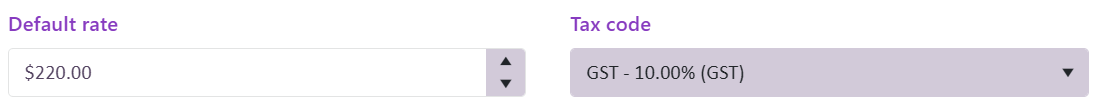Thus, when you add a new cost at the Activity List that is not linked to a particular category, it starts off with the system cost rate. You can alter this if desired.

The system cost rate is set at the Accounting tab of the system options, see System options.

## Rate rises and estimates

When you increase hourly rate(s) at the Activity List this does not automatically update exiting estimates and estimate templates.

There are three possibilities:

1. Estimate polices via activities (and contains activities)
2. The estimate costs are not automatically updated.

When you add a cost from the estimate the rate applied is the rate in the estimate, not the updated rate at the Activity List.

However, you can run the Estimate Rate Adjuster to update these kind of estimates.

3. Estimate polices via charge codes and contains charge codes
4. The estimate costs are not automatically updated.

When you add a cost from the estimate the rate applied is the updated rate at the Activity List.

The same amount of money is left because the estimate cost remains the same. Thus, the amount of time left decreases. See Rate rises and charge code-based estimates.

5. Estimate polices via charge codes and contains activities
6. The estimate costs are not automatically updated.

When you add a cost from the estimate the rate applied is the updated rate at the Activity List.

The same amount of money is left because the estimate cost remains the same. Thus, the amount of time left decreases.

Here the estimate costs can be updated using the Estimate Rate Adjuster. See Rate rises and charge code-based estimates.